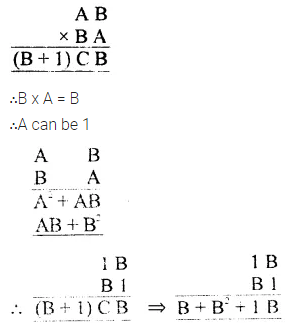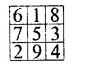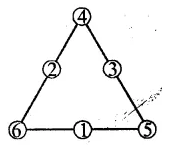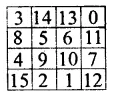# RS Aggarwal Class 8 Solutions Chapter 5 Playing with Numbers Ex 5C

## RS Aggarwal Class 8 Solutions Chapter 5 Playing with Numbers Ex 5C

These Solutions are part of RS Aggarwal Solutions Class 8. Here we have given RS Aggarwal Solutions Class 8 Chapter 5 Playing with Numbers Ex 5C.

Other Exercises

Replace A, B, C by suitable numerals.

Question 1.
Solution:
Here A can be as 6 + 7 = 13
Now 1 + 5 + 8 = 14
∴C = 1, B = 4, A = 6

Question 2.
Solution:
Here A can be 7, as 6+7 = 13
1 + B + 9 = 10 + B
∴B can be 7
∴10 + 7 = 17
1 + C + 6 = 7 + C
∴C can be 4
∴1 + 4 + 6 = 11
and 1 + 4 + 3 = 8
∴A = 7, B = 7, C = 4

Question 3.
Solution:
Here A + A + A = A
∴A can = 5
∴5 + 5 + 5 = 15
∴B = 1
Hence A = 5, B = 1

Question 4.
Solution:
6 – A = 3
1 + 5 – A = 3
5 – A = 3
∴A = 5 – 3 = 2
Now 2 – B = 7
=>12 – B = 7
∴B = 5
Hence A = 2, B = 5

Question 5.
Solution:
– 5 – A = 9 =>A = 5 – 9 or 15 – 9
= 6
∴A = 6
Now B – 1 – 8 = 5 =>B – 9 = 5
=>B = 5 + 9 = 14
∴B = 4
Now C – 1 – 2 = 2 =>C – 3 = 2
C = 2 + 3 = 5
∴A = 6, B = 4, C = 5

Question 6.
Solution:
B x 3 = B
∴B can be 5 or 0
∴5 x 3 = 15 => B = 5 or 3 x 0 =0
If B = 0, then A can be 5
∴3 x 5 = 15
∴A = 5 and C = 1
Hence A = 5, B = 0, C = 1

Question 7.
Solution:∴AB = B
=>A = 1
and A² + B² – 1 + B² + C
∴B² +1 = C
∴B² in one digit
If B = 3
∴3² + 1 = 9 + 1 = 10 = C
∴C = 0
B x 1 + 1 = B + 1 = 3 + 1
Hence A = 1, B = 3, C = 0

Question 8.
Solution:
Here we see that 6 x 9 = 54
∴A – 4 = 3 => A = 3 + 4 = 7
and 6 x 6 = 36
3B = 36 => B = 6
and C = 6
Hence A = 7, B = 6, C = 6

Question 9.
Solution:
Product of two numbers = 1 -digit number
and sum = 2-digit numbers
Let first number = x
and second number = y
∴x X y = 1-digit number
x + y = 2-digit number
By hit and hail, we sec that
1 x 9 = 9 which is I-digit number
and 1 + 9 = 10 which is 2-digit number

Question 10.
Solution:
By hit and trail method, we see that
1 + 2 + 3 = 6 and 1 x 2 x 3 = 6
1, 2 and 3 are the required whole numbers
whose sum and product is same

Question 11.
Solution:
In the given square, we have to interest the numbers from 1 to 9, such that the sum in each raw, column on diagonal to be 15
So, we complete it as given hereQuestion 12.
Solution:
We shall complete the triangle by intersecting the numbers from 1 to 6 without repetition so that the sum in each side be 12Question 13.
Solution:
The given numbers are
a, b (a + b), (a + 2b), (2a + 3b), (3a + 5b), (5a + 8b), (8a + 13b), (13a + 21b), and (21a + 34b)
Sum of there numbers = 11 (5a + 8b)
= 11 x 7th number
Now taking a = 8, b = 13, then the 10 number be 8, 13, 21, 34, 55, 89, 144, 233, 377, 610
Whose 7th number = 144
By adding these 10 numbers, we get the
sum
= 8+ 13 + 21 + 34 + 55 + 89 + 144 + 233 + 377 + 610 = 1584 and 11 x 7th number =11 x 144 , = 1584
Which is same in each case

Question 14.
Solution:
We see that in the magic box sum of 0 + 11 + 7 + 12 = 30
Now we shall complete this magic square, to get 30 as the sum in each row and column and also diagonal wiseHope given RS Aggarwal Solutions Class 8 Chapter 5 Playing with Numbers Ex 5C are helpful to complete your math homework.

If you have any doubts, please comment below. Learn Insta try to provide online math tutoring for you.